### Kinematic and Distributing Coupling

In this section the theoretical background of the keyword *COUPLING followed by *KINEMATIC or *DISTRIBUTING is covered, and not the keyword DISTRIBUTING COUPLING.

Coupling constraints generally lead to nonlinear equations. In linear calculations (without the parameter NLGEOM on the *STEP card) these equations are linearized once and solved. In nonlinear calculations, iterations are performed in each of which the equations are linearized at the momentary solution point until convergence.

Coupling constraints apply to all nodes of a surface given by the user. In a kinematic coupling constraint by the user specified degrees of freedom in these nodes follow the rigid body motion about a reference point (also given by the user). In CalculiX the rigid body equations elaborated in section 3.5 of  are implemented. Since CalculiX does not have internal rotational degrees of freedom, the translational degrees of freedom of an extra node (rotational node) are used for that purpose, cf. *RIGID BODY. Therefore, in the case of kinematic coupling the following equations are set up:

• 3 equations connecting the rotational degrees of freedom of the reference node to the translational degrees of freedom of an extra rotational node.
• per node belonging to the surface at stake, for each degree of freedom specified by the user (maximum 3) a rigid body equation.

This applies if no ORIENTATION was used on the *COUPLING card, i.e. the specified degrees of freedom apply to the global coordinate system. If an ORIENTATION parameter is used, the degrees of freedom apply in a local system. Then, the nodes belonging to the surface at stake (let us give them the numbers 1,2,3...) are duplicated (let us call these d1,d2,d3.....) and the following equations are set up:

• 3 equations connecting the rotational degrees of freedom of the reference node to the translational degrees of freedom of an extra rotational node.
• per duplicated node belonging to the surface at stake, a rigid body equation for each translational degree of freedom (i.e. 3 per duplicated node).
• per node an equation equating the by the user specified degrees of freedom in the local system (maximum 3) to the same ones in the duplicated nodes.

The approach for distributing coupling is completely different. Here, the purpose is to redistribute forces and moments defined in a reference node across all nodes belonging to a facial surface define on a *COUPLING card. No kinematic equations coupling the degrees of freedom of the reference node to the ones in the coupling surface are generated. Rather, a system of point loads equivalent to the forces and moments in the reference node is applied in the nodes of the coupling surface.

To this end the center of gravity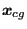of the coupling surface is determined by: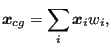(166)

where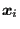are the locations of the nodes belonging to the coupling surface and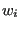are weights taking the area into account for which each of the nodes is “responsible”. We have: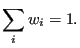(167)

The relative position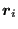of the nodes is expressed by: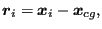(168)

and consequently: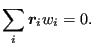(169)

The forces and momentsdefined by the user in the reference node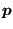can be transferred into an equivalent system consisting of the force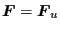and the moment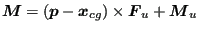in the center of gravity. Now, it can be shown by use of the above relations that the system consisting of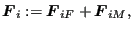(170)

where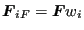(171)

and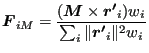(172)

using the definition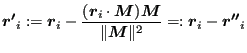(173)

is equivalent to the system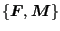in the center of gravity. The vector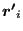is the orthogonal projection ofon a plane perpendicular to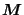. Notice that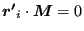and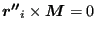.

The proof is done by calculating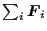and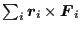and using the relationship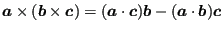. One obtains: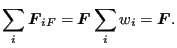(174)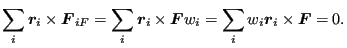(175)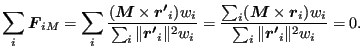(176)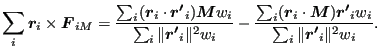(177)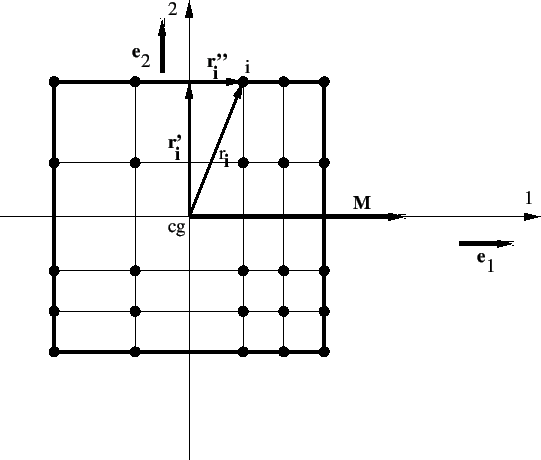The last equation deserves some further analysis. The first term on the right hand side amounts tosince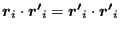. For the analysis of the second term a carthesian coordinate system consisting of the unit vectors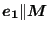,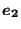and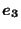is created (cf. Figure 126 for a 2-D surface in the 1-2-plane). The numerator of the second term amounts to: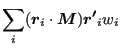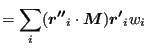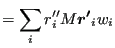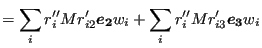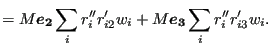(178)

These terms are zero (setting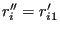) if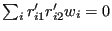and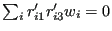i.e. if the carthesian coordinate system is parallel to the principal axes of inertia based on the weights. Consequently, for Eq. (172) to be valid,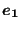,andhave to be aligned with the principal axes of inertia! The equivalent force and moment in the center of gravity are subsequently decomposed along these axes.

Defining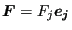and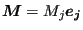one can write: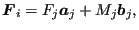(179)

where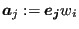(180)

and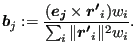(181)

Notice that the formula for the moments is the discrete equivalent of the well-known formulas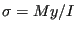for bending moments and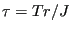for torques in beams .

Now, an equivalent formulation to Equation (179) for the user defined force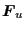and moment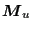is sought. In component notation Equation (179) runs: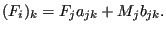(182)

Defining vectors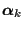and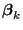such that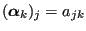and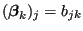this can be written as: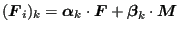(183)

or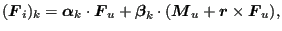(184)

where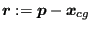. This is a linear function ofand: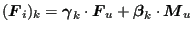(185)

where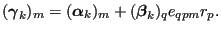(186)

The coefficients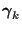andin Equation (185) are stored at the beginning of the calculation for repeated use in the steps (the forces and moments can change from step to step). Notice that the components ofandhave to be calculated in the local coupling surface coordinate system, whereas the result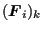applies in the global carthesian system.

If an orientation is defined on the *COUPLING card the force and moment contributions are first transferred into the global carthesian system before applying the above procedure. Right now, only carthesian local systems are allowed for distributing coupling.